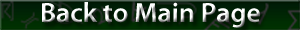Electrical Laws and Formulas

#### Electrical Laws and Formulas

Name Formula Notes
Ohm's Law E = IR E = voltage (in volts)
I = current (in amps)
R = resistance (in ohms)
Watt's Law P = IE P = power (in watts)
I = current (in amps)
E = voltage (in volts)
Joule's Law P = I2R P = power (in watts)
I = current (in amps)
R = resistance (in ohms)

Twelve formulas can be derived from the preceding laws, as shown here: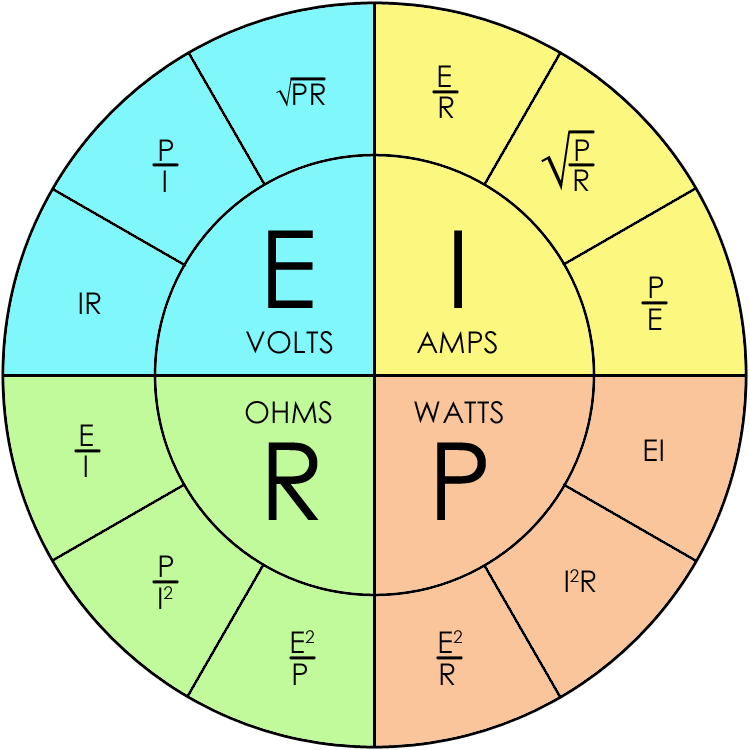Name Formula Notes
Resistance in a DC series circuit RT = R1 + R2 + R3 + ... + Rn R = resistance
Resistance in a DC parallel circuit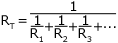R = resistance
Capacitance in a DC series circuit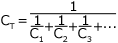C = capacitance
Capacitance in a DC parallel circuit CT = C1 + C2 + C3 + ... + Cn C = capacitance
Inductance in a DC series circuit LT = L1 + L2 + L3 + ... + Ln L = inductance
Inductance in a DC parallel circuit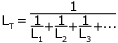L = inductance
Capacitive reactance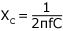f = frequency (in hertz)
Inductive reactance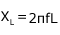f = frequency (in hertz)
L = inductance (in henries)
Resonance (XC = XL)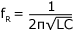fR = resonant frequency (in hertz)
L = inductance (in henries)
Admittance (reciprocal of impedance)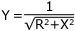Y = admittance (in mhos)
R = resistance (in ohms)
X = reactance (in ohms)
Susceptance (reciprocal of reactance)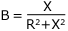B = susceptance (in mhos)
X = reactance (in ohms)
R = resitance in ohms)
Conductance (reciprocal of resistance)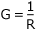G = conductance (in mhos)
R = resistance (in ohms)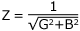or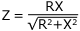Z = impedance (in ohms)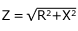Z = impedance (in ohms)
Frequency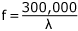f = frequency (in hertz)
Wavelength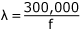f = frequency (in hertz)